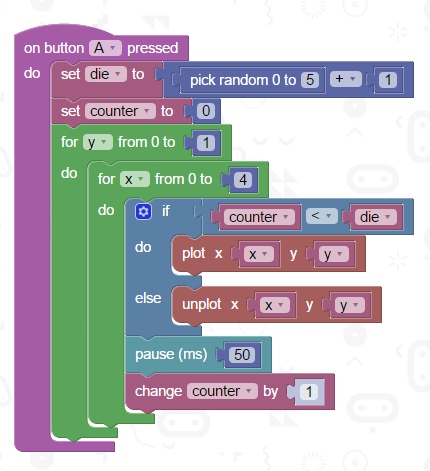# BBC micro:bit Two Dice

## Introduction

On one of the previous pages, you might have been making a program to turn the micro:bit into a die that you could 'roll' by shaking the micro:bit. Here is one way to do that whenever the user pressed the A button.We don't get a choice about the bottom range of the random numbers. So we generate a number from 0-5 and then add one. The loops then plots as many pixels on the matrix as the number on the die.

## Two Dice

Rolling two dice, and adding them together, we get a number from 2 to 12. The lowest we can roll is double 1, making a total of 2. The highest we can roll is a double 6, making a total of 12. Extending the logic of the previous program, we might foolishly do this,This will make between 2 and 12 dots on the screen each time. It isn't right though. Over time, the random number generator generates numbers that are distributed evenly across the range. That doesn't happen with two dice. There is only one way to make 12 and there are 6 ways to make a total of 7. The quickest fix for that is to make the program get the total from two random dice,## Challenges

1. 3 Dice.
2. Use the display logic for some other purpose. Maybe you want to be able to read a number from 1 to 25 from the matrix without having to have it scroll when in the two digit range.